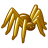# K70 DDR2 read failure with increasing temperature

cancel
Showing results for
Did you mean:

## K70 DDR2 read failure with increasing temperature

1,383 ViewsContributor II

Hello everybody.

We are using K70 controller (MK70FN1M0VMJ15 rev. 3N96B).

Architecture is the same as the tower TWRK70F120 supplied by NXP.

To reproduce the issue, we are running a simple test software that continues to write and read different values (0x00 to 0xff) in a loop into the whole 128 MB external RAM memory (Samsung K4T1G164QG-BCE7).

Once the board is heated to 40 °C or more, the RAM test fails. Note that once the problem arises, the faulty reading persists with following retries.

Our software is using MQX 4.1.0 operating system and the DDR controller is initialized by means of _bsp_ddr2_setup provided in init_hw.c (BSP library) module, as it is, without changes:

void _bsp_ddr2_setup (void)
{
SIM_MemMapPtr sim = SIM_BASE_PTR;
DDR_MemMapPtr ddr = DDR_BASE_PTR;
MCM_MemMapPtr mcm = MCM_BASE_PTR;

/* Enable DDR controller clock */

/* Enable DDR pads and set slew rate */
sim->MCR |= 0xC4; /* bits were left out of the manual so there isn't a macro right now */

* (volatile uint32_t *)(0x400Ae1ac) = 0x01030203;

/* TC's init */
ddr->CR00 = 0x00000400;
ddr->CR02 = 0x02000031;
ddr->CR03 = 0x02020506;
ddr->CR04 = 0x06090202;
ddr->CR05 = 0x02020302;
ddr->CR06 = 0x02904002;
ddr->CR07 = 0x01000303;
ddr->CR08 = 0x05030201;
ddr->CR09 = 0x020000c8;
ddr->CR10 = 0x03003207;
ddr->CR11 = 0x01000000;
ddr->CR12 = 0x04920031;
ddr->CR13 = 0x00000005;
ddr->CR14 = 0x00C80002;
ddr->CR15 = 0x00000032;
ddr->CR16 = 0x00000001;
ddr->CR20 = 0x00030300;
ddr->CR21 = 0x00040232;
ddr->CR22 = 0x00000000;
ddr->CR23 = 0x00040302;
ddr->CR25 = 0x0A010201;
ddr->CR26 = 0x0101FFFF;
ddr->CR27 = 0x01010101;
ddr->CR28 = 0x00000003;
ddr->CR29 = 0x00000000;
ddr->CR30 = 0x00000001;
ddr->CR34 = 0x02020101;
ddr->CR36 = 0x01010201;
ddr->CR37 = 0x00000200;
ddr->CR38 = 0x00200000;
ddr->CR39 = 0x01010020;
ddr->CR40 = 0x00002000;
ddr->CR41 = 0x01010020;
ddr->CR42 = 0x00002000;
ddr->CR43 = 0x01010020;
ddr->CR44 = 0x00000000;
ddr->CR45 = 0x03030303;
ddr->CR46 = 0x02006401;
ddr->CR47 = 0x01020202;
ddr->CR48 = 0x01010064;
ddr->CR49 = 0x00020101;
ddr->CR50 = 0x00000064;
ddr->CR52 = 0x02000602;
ddr->CR53 = 0x03c80000;
ddr->CR54 = 0x03c803c8;
ddr->CR55 = 0x03c803c8;
ddr->CR56 = 0x020303c8;
ddr->CR57 = 0x01010002;

_ASM_NOP();

ddr->CR00 |= 0x00000001;

while ((ddr->CR30 & 0x400) != 0x400) {
}

mcm->CR |= MCM_CR_DDRSIZE(1);
}

Not all the boards are experiencing the problem, but roughly 60 % of them.

We suspect that the issue may be related to the K70 DDR controller.

We tried to apply the advice in this document (erratum ID e10521), and tried also various RCR values other than those read by the procedure explained in e10521, but with no success.

Update 2017-04-07:

We tried to apply a different ddr2 setup by using the output produced by Freescale's K70memctrl (we found it here) with the following command:

K70memctrl c MT47H64M16.mem ddr2setup.c

and reporting the output of ddr2setup.c in our initialization function:

void _bsp_ddr2_setup_modified (void)
{
SIM_MemMapPtr sim = SIM_BASE_PTR;
DDR_MemMapPtr ddr = DDR_BASE_PTR;
MCM_MemMapPtr mcm = MCM_BASE_PTR;

/* Enable DDR controller clock */

/* Enable DDR pads and set slew rate */
sim->MCR |= 0xC4; /* bits were left out of the manual so there isn't a macro right now */

* (volatile uint32_t *)(0x400Ae1ac) = 0x01030203;

/* TC's init */
ddr->CR00 = 0x00000400;
ddr->CR02 = 0x02007530;
ddr->CR03 = 0x02020707;
ddr->CR04 = 0x07090202;
ddr->CR05 = 0x02020302;
ddr->CR06 = 0x00290402;
ddr->CR07 = 0x01010303;
ddr->CR08 = 0x06030301;
ddr->CR09 = 0x020000c8;
ddr->CR10 = 0x02000808;
ddr->CR11 = 0x01000000;
ddr->CR12 = 0x048a001e;
ddr->CR13 = 0x00000005;
ddr->CR14 = 0x00c70002;
ddr->CR15 = 0x00000015;
ddr->CR16 = 0x00000001;
ddr->CR20 = 0x00030300;
ddr->CR21 = 0x24040232;
// ddr->CR22 = 0x00000000;
// ddr->CR23 = 0x00040302;
ddr->CR25 = 0x0A010201;
ddr->CR26 = 0x0101FFFF;
ddr->CR27 = 0x00010101;
ddr->CR28 = 0x00000001;
// ddr->CR29 = 0x00000000;
ddr->CR30 = 0x00000001;
ddr->CR34 = 0x00000101;
// ddr->CR36 = 0x01010201;
ddr->CR37 = 0x00000200;
ddr->CR38 = 0x00200000;
ddr->CR39 = 0x00000020;
ddr->CR40 = 0x00002000;
ddr->CR41 = 0x01010020;
ddr->CR42 = 0x00002000;
ddr->CR43 = 0x02020020;
// ddr->CR44 = 0x00000000;
ddr->CR45 = 0x00070b0f;
ddr->CR46 = 0x0f004000;
ddr->CR47 = 0x0100070b;
ddr->CR48 = 0x0b0f0040;
ddr->CR49 = 0x00020007;
ddr->CR50 = 0x00000040;
ddr->CR52 = 0x02000602;
// ddr->CR53 = 0x03c80000;
// ddr->CR54 = 0x03c803c8;
// ddr->CR55 = 0x03c803c8;
ddr->CR56 = 0x02030000;
ddr->CR57 = 0x01000000;

_ASM_NOP();

ddr->CR00 |= 0x00000001;

while ((ddr->CR30 & 0x400) != 0x400) {
}

mcm->CR |= MCM_CR_DDRSIZE(1);
}

With this setup, the problem on faulty boards is occurring much less often within a series of tests, but it is always present.

Any hints?

Labels (2)

• ### Kinetis K Series MCUs

Tags (3)
3 Replies
756 ViewsContributor II

For those who may be interested in, finally we found that although there may be other settings for CRnn registers that we need to fix, depending on our memory chip, using the Kinetis K70 DDR memory initialization tool (ARM Cortex-M4|Kinetis K70 120-150 MHz 32-bit MCUs|NXP), what really is needed is adding a clear of some bits in the MCR:

sim->MCR &= 0xFFFFFF00;

before the OR operation in MQX initialization:

sim->MCR |= 0xC4;

It turned out that the DDRDQSDIS bit (3rd bit) is 1 at MCU reset (Configure the DDR_DQS pins in a low power state!!!) and this is documented only in Rev. 3 of the reference manual, while according to Rev. 2 it should have been 0.

756 ViewsContributor I

We also found the same problem, sim->MCR &= 0xFFFFFF00; (+80 c) after the test is normal, but (-10c) near the same problem, your side for this test?

I found DDRDQSDIS = 1 high temperature ddr data error, DDRDQSDIS = 0 low temperature(-10C) error

Finally, I by single chip microcomputer internal ADC temperature sensor, automatic adjustment, but long run time is still likely to collapse

Is there a better solution?

756 ViewsSenior Contributor III

Fast Accurate Memory Test Code in C | Barr Group in the comments they note about DDR2 issues of power and cross talk showing up with 0xFF/0x00 type tests.  Issues may exist at all temperatures just appear at the higher ones.